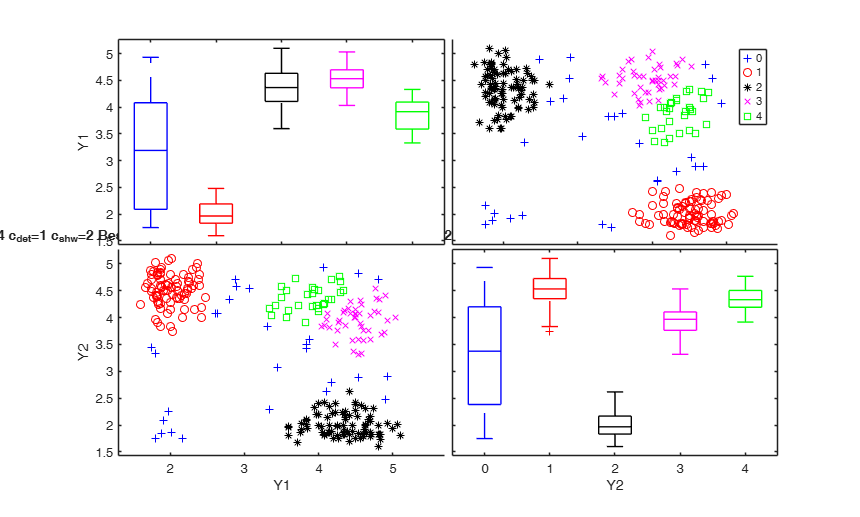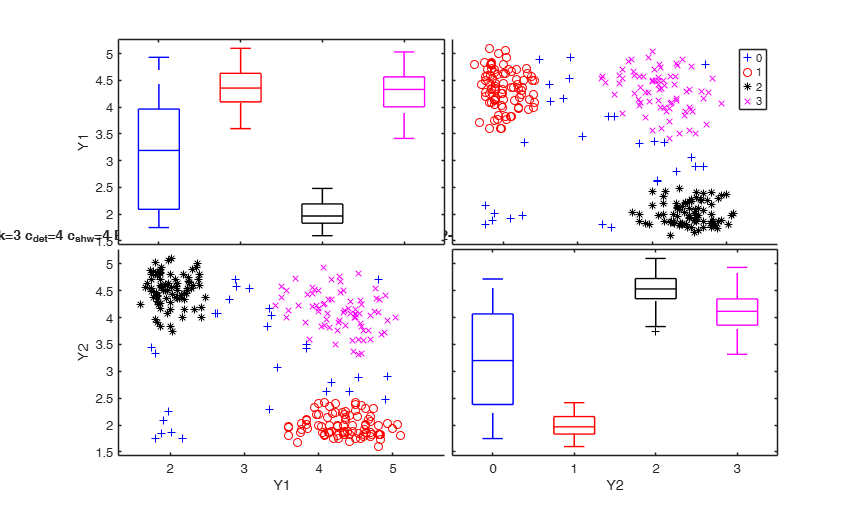tclustICsolGPCM

tclustICsolGPCM extracts a set of best relevant solutions from 3D array computed using function tclustICgpcm

Syntax

• out=tclustICsolGPCM(IC)example
• out=tclustICsolGPCM(IC,Name,Value)example

Description

tclustICsolGPCM takes as input the output of function tclustICgpcm that is a series of matrices which contain the values of the information criteria BIC/ICL/CLA for different values of $k$ and $c_{det}$ and $c_{shw}$ (for fixed trimming level $\alpha$) and extracts the first best solutions. Two solutions are considered equivalent if the value of the adjusted Rand index (or the adjusted Fowlkes and Mallows index) is above a certain threshold. For each tentative solution the program checks the adjacent values of $c_{det}$ and $c_{shw}$ for which the solution is stable. A matrix with adjusted Rand indexes is given for the extracted solutions.

out =tclustICsolGPCM(IC) Plot of first two best solutions for Geyser data.

out =tclustICsolGPCM(IC, Name, Value) Simulated data: compare first 2 best solutions using MIXMIX and CLACLA.

Examples

expand allPlot of first two best solutions for Geyser data.

% nsamp=30 to reduce computational time
outIC=tclustICgpcm(Y,'cleanpool',false,'plots',0,'alpha',0.1,'nsamp',30);
% Plot first two best solutions using as Information criterion MIXMIX
disp('Best solutions using MIXMIX')
[out]=tclustICsolGPCM(outIC,'whichIC','MIXMIX','plots',1,'NumberOfBestSolutions',2);
disp(out.MIXMIXbs)
k=1
k=2
k=3
k=4
k=5
Best solutions using MIXMIX
Columns 1 through 5

{}    {}    {[2 4 8 16 32 64 128]}    {[       1]}    {'true'    }
{}    {}    {[1 2 4 8 16 32 64 128]}    {0×0 double}    {'spurious'}

Columns 6 through 8

{}    {[2 4 8 16 32 64 128]}    {}
{}    {[2 4 8 16 32 64 128]}    {}Simulated data: compare first 2 best solutions using MIXMIX and CLACLA.

Data generation

restrfact=5;
rng('default') % Reinitialize the random number generator to its startup configuration
rng(10000);
ktrue=3;
% n = number of observations
n=150;
% v= number of dimensions
v=2;
% Imposed average overlap
BarOmega=0.04;
outMS=MixSim(ktrue,v,'BarOmega',BarOmega, 'restrfactor',restrfact);
% data generation given centroids and cov matrices
[Y,id]=simdataset(n, outMS.Pi, outMS.Mu, outMS.S);
% Specify number of solutions
NumberOfBestSolutions=2;
% Number of subsets to extract
nsamp=100;
% Computation of information criterion using MIXMIX
outICmixt=tclustICgpcm(Y,'plots',0,'nsamp',nsamp);
% Plot first 2 best solutions using as Information criterion MIXMIX
disp('Best 2 solutions using MIXMIX')
[outMIXMIX]=tclustICsolGPCM(outICmixt,'whichIC','MIXMIX','plots',1,'NumberOfBestSolutions',NumberOfBestSolutions);
disp(outMIXMIX.MIXMIXbs)
% Computation of information criterion using CLACLA
outICcla=tclustICgpcm(Y,'whichIC','CLACLA','plots',0,'nsamp',nsamp);
[outCLACLA]=tclustICsolGPCM(outICcla,'whichIC','CLACLA','plots',1,'NumberOfBestSolutions',NumberOfBestSolutions);
disp('Best 2 solutions using CLACLA')
disp(outCLACLA.CLACLAbs)

Related Examples

expand all

An example with input options Rand and kk.

nsamp=100;
pa=struct;
pa.cdet=[2 4];
pa.shw=[8 16 32];
kk=[2 3 4 6];
out=tclustICgpcm(Y,'pa',pa,'cleanpool',false,'plots',0,'alpha',0.1,'whichIC','CLACLA','kk',kk,'nsamp',nsamp);
[outCLACLA]=tclustICsolGPCM(out,'whichIC','CLACLA','plots',1,'NumberOfBestSolutions',3,'Rand',0);

Input Arguments

IC — Information criterion to use. Structure.

It contains the following fields.

Value Description
CLACLA

3D array of size length(kk)-by-length(cdet)-by-length(cshw) containinig the values of the penalized classification likelihood (CLA).

This field is linked with IC.IDXCLA.

IDXCLA

3D array of size length(kk)-by-length(cdet)-by-length(csshw).

Each element of the cell is a vector of length n containinig the assignment of each unit using the classification model.

Remark: fields CLACLA and IDXCLA are linked together.

CLACLA and IDXCLA are compulsory just if optional input argument 'whichIC' is 'CLACLA'.

MIXMIX

3D array of size length(kk)-by-length(cdet)-by-length(cshw) containinig the value of the penalized mixture likelihood (BIC). This field is linked with IC.IDXMIX.

MIXCLA

3D array of size length(kk)-by-length(cdet)-by-length(cshw) containinig the value of the ICL. This field is linked with IC.IDXMIX.

IDXMIX

3D cell of size length(kk)-by-length(cdet)-by-length(cshw).

Each element of the cell is a vector of length n containinig the assignment of each unit using the mixture model.

Remark 1: fields MIXMIX and IDXMIX are linked together.

MIXMIX and IDXMIX are compulsory just if optional input argument 'whichIC' is 'CLACLA'.

Remark 2: fields MIXCLA and IDXMIX are linked together.

MIXCLA and IDXMIX are compulsory just if optional input argument 'whichIC' is 'MIXCLA'.

kk

vector containing the values of k (number of components) which have been considered.

ccdet

vector containing the values of cdet (values of the restriction factor for ratio of determinants) which have been considered.

ccshw

vector containing the values of cshw (values of the restriction factor for ratio of elements of shape matrices inside each group) which have been considered.

alpha

scalar containing the values of trimming level which has been considered.

Y

original n-times-v data matrix on which the IC (Information criterion) has been computed. This input option is present only if IC comes from tclustIC.

Data Types: struct

Name-Value Pair Arguments

Specify optional comma-separated pairs of Name,Value arguments. Name is the argument name and Value is the corresponding value. Name must appear inside single quotes (' '). You can specify several name and value pair arguments in any order as Name1,Value1,...,NameN,ValueN.

Example: 'NumberOfBestSolutions',5 , 'ThreshRandIndex',0.8 , 'whichIC','CLACLA' , 'plots',1 , 'SpuriousSolutions',false , 'msg',1 , 'Rand',1

NumberOfBestSolutions —number of solutions to consider.scalar integer greater than 0.

Number of best solutions to extract from BIC/ICL matrix. The default value of NumberOfBestSolutions is 5

Example: 'NumberOfBestSolutions',5

Data Types: int16 | int32 | single | double

ThreshRandIndex —threshold to identify spurious solutions.positive scalar between 0 and 1.

Scalar which specifies the threshold of the adjusted Rnd index to use to consider two solutions as equivalent. The default value of ThreshRandIndex is 0.7

Example: 'ThreshRandIndex',0.8

Data Types: single | double

whichIC —character which specifies the information criterion to use to extract best solutions.character.

Possible values for whichIC are:

'CLACLA' = in this case best solutions are referred to the classification likelihood.

'MIXMIX' = in this case best solutions are referred to the mixture likelihood (BIC).

'MIXCLA' = in this case best solutions are referred to ICL.

The default value of 'whichIC' is 'MIXMIX'

Example: 'whichIC','CLACLA'

Data Types: character

plots —plots of best solutions on the screen.scalar.

It specifies whether to plot on the screen the best solutions which have been found.

Example: 'plots',1

Data Types: single | double

SpuriousSolutions —Include or nor spurious solutions in the plot.boolean.

As default spurios solutions are shown in the plot.

Example: 'SpuriousSolutions',false

Data Types: single | double

msg —Message on the screen.scalar.

Scalar which controls whether to display or not messages about code execution.

The default value of msg is 0, that is no message is displayed on the screen.

Example: 'msg',1

Data Types: single | double

Rand —Index to use to compare partitions.scalar.

If Rand =1 (default) the adjusted Rand index is used, else the adjusted Fowlkes and Mallows index is used

Example: 'Rand',1

Data Types: single | double

Output Arguments

out — description Structure

Structure which contains the following fields:

Value Description
MIXMIXbs

cell of size NumberOfBestSolutions-times-8 which contains the details of the best solutions for MIXMIX (BIC).

Each row refers to a solution. The information which is stored in the columns is as follows.

1st col = scalar, value of k for which solution takes place;

2nd col = scalar, value of cdet for which solution takes place;

3rd col = row vector of length d which contains the values of cdet for which the solution is uniformly better.

4th col = row vector of length d+r which contains the values of cdet for which the solution is considered stable (i.e. for which the value of the adjusted Rand index, or the adjusted Fowlkes and Mallows index) does not go below the threshold defined in input option ThreshRandIndex).

5th col = string which contains 'true' or 'spurious'. The solution is labelled spurious if the value of the adjusted Rand index with the previous solutions is greater than ThreshRandIndex.

6th col = scalar, value of cshw for which solution takes place.

7th col = row vector of length d which contains the values of cshw for which the solution is uniformly better.

8th col = row vector of length d+r which contains the values of cshw for which the solution is considered stable (i.e. for which the value of the adjusted Rand index, or the adjusted Fowlkes and Mallows index) does not go below the threshold defined in input option ThreshRandIndex).

Remark: field out.MIXMIXbs is present only if input option 'whichIC' is 'MIXMIX'.

MIXMIXbsari

matrix of adjusted Rand indexes (or Fowlkes and Mallows indexes) associated with the best solutions for MIXMIX. Matrix of size NumberOfBestSolutions-times-NumberOfBestSolutions whose i,j-th entry contains the adjusted Rand (or Fowlkes and Mallows) index between classification produced by solution i and solution j, $i,j=1, 2, \ldots, NumberOfBestSolutions$.

Remark: field out.MIXMIXbsari is present only if 'whichIC' is 'MIXMIX'.

MIXCLAbs

this output has the same structure as out.MIXMIXbs but it is referred to MIXCLA.

Remark: field out.MIXCLAbs is present only if 'whichIC' is 'MIXCLA'.

MIXCLAbsari

this output has the same structure as out.MIXMIXbs but it is referred to MIXCLA.

Remark: field out.MIXCLAbsari is present only if 'whichIC' is 'MIXCLA'.

CLACLAbs

this output has the same structure as out.MIXMIXbs but it is referred to CLACLA.

Remark: field out.CLACLAbs is present only if 'whichIC' is 'CLACLA'.

CLACLAbsari

this output has the same structure as out.MIXMIXbs but it is referred to CLACLA.

Remark: field out.MIXCLAbsari is present only if 'whichIC' is 'ALL' or 'whichIC' is 'CLACLA'

MIXCLAbsIDX

matrix of dimension n-by-NumberOfBestSolutions containing the allocations for MIXCLA associated with the best NumberOfBestSolutions. This field is present only if 'whichIC' is 'MIXCLA'.

MIXMIXbsIDX

matrix of dimension n-by-NumberOfBestSolutions containing the allocations for MIXMIX associated with the best NumberOfBestSolutions. This field is present only if 'whichIC' is 'MIXMIX'.

CLACLAbsIDX

matrix of dimension n-by-NumberOfBestSolutions containing the allocations for CLACLA associated with the best NumberOfBestSolutions. This field is present only if 'whichIC' is 'CLACLA'.

kk

vector containing the values of k (number of components) which have been considered. This vector is equal to input optional argument kk if kk had been specified else it is equal to 1:5.

ccdet

vector containing the values of cdet (values of the restriction factor for determinants) which have been considered. This vector is equal to input argument IC.cdet.

ccshw

vector containing the values of cshw (values of the restriction factor for shape elements inside each group) which have been considered. This vector is equal to input argument IC.cshw.

alpha

scalar containing the value of $\alpha$ (trimming level) which have been considered. This output is equal to input argument IC.alpha.

Cerioli, A., Garcia-Escudero, L.A., Mayo-Iscar, A. and Riani M. (2017), Finding the Number of Groups in Model-Based Clustering via Constrained Likelihoods, "Journal of Computational and Graphical Statistics", pp. 404-416, https://doi.org/10.1080/10618600.2017.1390469

Hubert L. and Arabie P. (1985), Comparing Partitions, "Journal of Classification", Vol. 2, pp. 193-218.